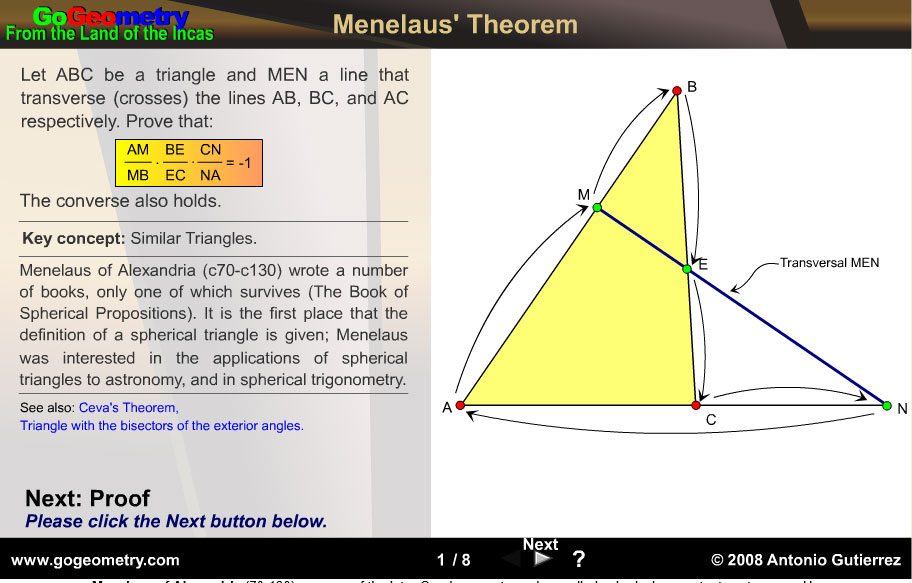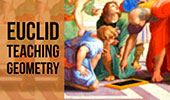Menelaus of Alexandria (70-130) was one of the later Greek geometers who applied spherical geometry to astronomy. He is best known for the so-called Menelaus's theorem.

 Online Geometry, See also: Ceva's Theorem. Concurrency. Interactive proof with animation. Puzzle of the Ceva's Theorem: 48 classic piece. Nagel Point Theorem. Proof. Gergonne Point Theorem. Concurrency. Interactive proof with animation. Key concept: Ceva's Theorem.Monge & d'Alembert Three Circles Theorem II with Dynamic Geometry Newton's Theorem: Newton's Line. Circumscribed quadrilateral, midpoints of diagonals, center of the circle inscribed. Puzzle of the Newton's Theorem: 50 pieces of circles. Pentagons & Pentagrams. I found, exploring with dynamic geometry software, new facts about pentagons and pentagrams. Key concept: Menelaus Theorem. See also: Pentagons & Pentagrams - Puzzle: 96 triangles. Eyeball Theorem: Animated Angle to Geometry Study. Geometry Problem 659. Parallelogram, Parallel Lines, Collinear Points.Home | Sitemap | Search | Geometry | Classical Theorems | Adobe Flash | Transversal | Email | By Antonio Gutierrez